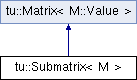CMR  1.3.0
tu::Submatrix< M > Class Template Reference

#include <matrix.hpp>

Inheritance diagram for tu::Submatrix< M >:## Public Types

typedef M::Index Index

typedef M::Value Value

typedef M::Nonzero NonzeroPublic Types inherited from tu::Matrix< M::Value >
typedef M::Value Value

typedef std::size_t Index
Type of entries. More...

## Public Member Functions

Submatrix (const M &matrix, const SubmatrixIndices &indices)
Constructs submatrix of matrix indexed by indices. More...

Submatrix (Submatrix &&other)
Move constructor. More...

std::size_t numRows () const
Returns the number of rows. More...

std::size_t numColumns () const
Returns the number of columns. More...

bool hasSortedRows () const
Indicates if the row data is sorted by column. More...

bool hasSortedColumns () const
Indicates if the column data is sorted by row. More...

const Valueget (Index row, Index column) const
Returns entry at row, column. More...

void ensureConsistency () const
Checks for consistency, raising a std::runtime_error if inconsistent. More...

## Member Typedef Documentation

template<typename M >
 typedef M::Index tu::Submatrix< M >::Index
template<typename M >
 typedef M::Nonzero tu::Submatrix< M >::Nonzero
template<typename M >
 typedef M::Value tu::Submatrix< M >::Value

## Constructor & Destructor Documentation

template<typename M >
 tu::Submatrix< M >::Submatrix ( const M & matrix, const SubmatrixIndices & indices )
inline

Constructs submatrix of matrix indexed by indices.

template<typename M >
 tu::Submatrix< M >::Submatrix ( Submatrix< M > && other )
inline

Move constructor.

## Member Function Documentation

template<typename M >
 void tu::Submatrix< M >::ensureConsistency ( ) const
inline

Checks for consistency, raising a std::runtime_error if inconsistent.

template<typename M >
 const Value& tu::Submatrix< M >::get ( Index row, Index column ) const
inline

Returns entry at row, column.

Returns entry at row, column. If the row or column data is sorted (resp. not sorted) then the time is logarithmic (resp. linear) in the number of nonzeros of the row or column.

template<typename M >
 bool tu::Submatrix< M >::hasSortedColumns ( ) const
inline

Indicates if the column data is sorted by row.

template<typename M >
 bool tu::Submatrix< M >::hasSortedRows ( ) const
inline

Indicates if the row data is sorted by column.

template<typename M >
 std::size_t tu::Submatrix< M >::numColumns ( ) const
inline

Returns the number of columns.

template<typename M >
 std::size_t tu::Submatrix< M >::numRows ( ) const
inline

Returns the number of rows.

The documentation for this class was generated from the following file:
• /home/travis/build/discopt/cmr/src/cmr/matrix.hpp